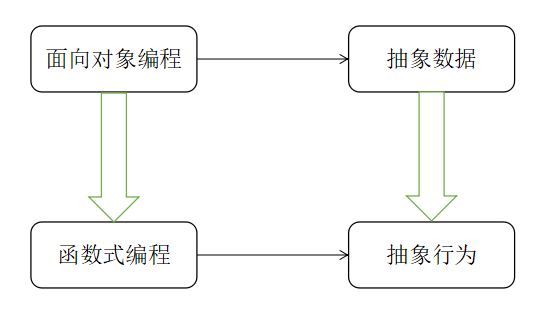# 走近函数式编程

0

## 活动信息

• 时间：2019.03.30 14:00 - 17:00
• 地点：成都市高新区世纪城路 1029 号天华社区乡愁故事馆

## 活动流程

14:00 Who are you?
14:10 函数式编程之我知
14:40 函数式编程之我用
16:00 你谈？我谈？共交流

## 活动总结

### What ?

``````function 煮(肉) {
return 水 + 火 + 肉;
}

function 炒(...食材) {
return 油 + 火 + 食材 + 盐;
}

let 回锅肉 = 炒(炒(切(煮(肉))), 切(蒜苗), 豆豉);
``````### Why & How ?

1. 方便单元测试
2. 减少外部状态干扰
3. 通过高阶抽象方便阅读、灵活组合

``````//  罗马数字与阿拉伯数字的对应
const roman_arab = {
I: 1,
V: 5,
X: 10,
L: 50,
C: 100,
D: 500,
M: 1000
};
``````

• I 可在 V、X 前，表减 1（如 IV 表示 4）
• X 可在 L、C 前，表减 10
• C 可在 D、M 前，表减 100

``````const romanLetterToInt = (letter) => {
const table = {
'I': 1,
'V': 5,
'X': 10,
'L': 50,
'C': 100,
'D': 500,
'M': 1000
}
if (!table[letter]) {
throw Error('capacity should be positive integer')
} else {
return table[letter];
}
}

const strSplit = (str) => {
return str.split('').map(romanLetterToInt);
}

const subtractItem = (arr) => {
return arr.map((element, index, arr) => {
return index < arr.length - 1 && element < arr[index + 1] ? -element : element
})
}

const getInt = (str) => {
return subtractItem(strSplit(str)).reduce((acc, curr) => {
return acc += curr;
},0)
}
``````

``````const table = {
5: ['I', 'V', 'X'],
50: ['X', 'L', 'C'],
500: ['C', 'D', 'M']
}

const intToIString = (number) => {
return new Array(number).fill('I').join('');
}

const mergeLetter = (str, numIndex) => {
const length = str.length;
const headLen = Math.floor(length / 10);
const tailLen = length % 10;
switch (tailLen) {
case 9:
case 4:
default:
const tailArray = new Array(Math.floor(tailLen / 5)).fill(table[numIndex]).concat(str.substring(str.length - tailLen % 5).split(''));
}
}

const splitStr = (str, numIndex) => {
const index = str.split('').findIndex((ele) => {
return ele !== table[numIndex];
})
return index >= 0 ? [str.substring(0, index), str.substring(index)] : [str, ''];
}

const mergeStr = (arr, numIndex) => {
return mergeLetter(arr, numIndex) + arr;
}

const getRoman = (num) => {
return mergeStr(splitStr(mergeStr(splitStr(mergeStr(splitStr(intToIString(num), 5), 5), 50), 50), 500), 500);
}
``````

### 再举个栗子

1. 每局比赛每人有十轮投球
2. 每轮共有两次机会来打倒全部十个瓶子
3. 一次打完为“全中”，本轮得分为 10 + 后两球分数
4. 两次打完为“补中”，本轮得分为 10 + 后一球分数
5. 第十轮全中、补中加 2、1 次击球

(下局第一次击球分数 + 下局第二次击球分数) x 全中系数

``````//  两数相加
function sum(first, second) {
return first + second;
}

//  补中系数
function isSpare(first, second) {
return first !== 10 && first + second === 10 ? 1 : 0;
}

//  全中系数
function isStrike(first) {
return first === 10 ? 1 : 0;
}

//  每轮分数
function round(this_first, this_second, next_one, next_two) {
return (
this_first +
this_second +
isSpare(this_first, this_second) * next_one +
isStrike(this_first, this_second) * (next_one + next_two)
);
}
``````

``````function curry(origin) {
//  原函数声明了几个参数
const count = origin.length;
//  包装函数
return function wrapper() {
//  当前是否已有足够的参数
return count > arguments.length
? //  还差几个，返回一个记住已传入参数的新函数
wrapper.bind(this, ...arguments)
: //  够了，执行原函数
origin.apply(this, arguments);
};
}
``````

``````const curry_round = curry(round),
score = [];

score = curry_round(3)(7)(3)(4); // 13

score = curry_round(3)(4)(0)(0); // 7

score = curry_round(10)(0)(3)(7); // 20

//  以此类推……
``````

## 活动反馈

1. 编辑器居然没有设置自动保存
2. 不写注释一时爽，一直不写一直爽
3. 其实讲一讲 Redux，对大家理解函数式编程帮助比较大
4. 其实讲一些实用的例子会更好，也更容易理解！
5. 听了对柯里化理解还不够
6. 罗马数字转阿拉伯数字的例子不错。阿拉伯数字转罗马数字的例子可以不用讲了，比较冗余了。可以加点例子: 如果其中某个函数抛出异常了怎么处理。

## 剪影1赞